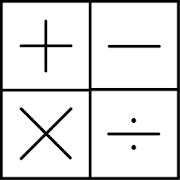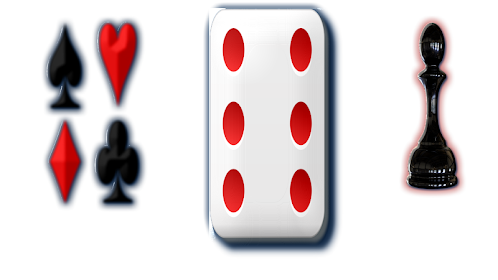# SuperMath FantogameEveryone
goal of the game reach a 3-digit number with 5 moves that consist of the use of one of 4 operations
more delete a digit of only one number.

operations: addition, subtraction, multiplication and division.
more delete a digit of one of 2 number.

how to play of the game:

at the beginning of the game are given:
a starting number of 3 digits (total)
the number to reach, always 3 digits.

for each round:

at the beginning of the round an additional 2-digit number is assigned (operand)

1) can remove a digit from one from operands or from total (optional)

2) sum or subtract or multiply or divide the total and operand:

subtract is always total minus operand
divide is always total divide operand

3) the result will be the new total

after 5th round the play ends.

the score categories:

gold:
if,in any of 5 rounds, the total is equals with the target number (in that case the play ends)

silver:
if, after the 5th round, the total is near with the target number
bronze:
if, after the 5th round, the total is least near with the target number

the score categories range are always showed at start of play

the number target is showed in gold color (yellow)

in the online multiplayer, the numbers: target, start total and all operands are the same for everyone and the one who gets more closer to the goal wins
Collapse

Review Policy

## What's New

Multiplayer not working
Collapse

Updated
February 15, 2019
Size
27M
Installs
10+
Current Version
1.0
Requires Android
4.1 and up
Content Rating
Everyone
Permissions
Offered By
Gabriele Fantoni
Developer
Gabriele Fantoni via prospero finzi 38 milano italiaSee more